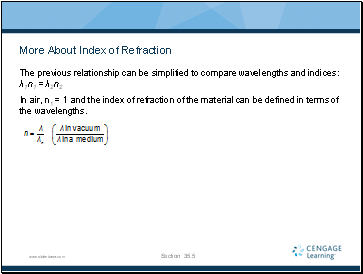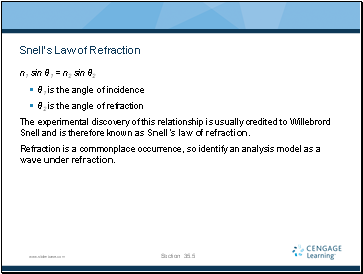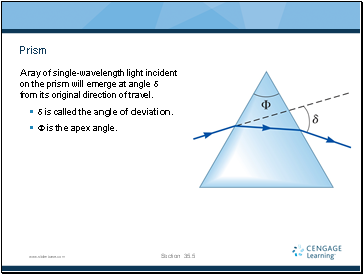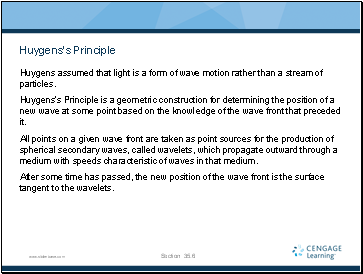# The Nature of Light and the Laws of Geometric OpticsPage 6

#### WATCH ALL SLIDES

The ratio of the indices of refraction of the two media can be expressed as various ratios.

The index of refraction is inversely proportional to the wave speed.

As the wave speed decreases, the index of refraction increases.

The higher the index of refraction, the more it slows downs the light wave speed.

Section 35.5

Slide 36## More About Index of Refraction

The previous relationship can be simplified to compare wavelengths and indices: λ1n1 = λ2n2

In air, n1 = 1 and the index of refraction of the material can be defined in terms of the wavelengths.

Section 35.5

Slide 37## Snell’s Law of Refraction

n1 sin θ1 = n2 sin θ2

θ1 is the angle of incidence

θ2 is the angle of refraction

The experimental discovery of this relationship is usually credited to Willebrord Snell and is therefore known as Snell’s law of refraction.

Refraction is a commonplace occurrence, so identify an analysis model as a wave under refraction.

Section 35.5

Slide 38## Prism

A ray of single-wavelength light incident on the prism will emerge at angle d from its original direction of travel.

d is called the angle of deviation.

F is the apex angle.

Section 35.5

Slide 39## Huygens’s Principle

Huygens assumed that light is a form of wave motion rather than a stream of particles.

Huygens’s Principle is a geometric construction for determining the position of a new wave at some point based on the knowledge of the wave front that preceded it.

All points on a given wave front are taken as point sources for the production of spherical secondary waves, called wavelets, which propagate outward through a medium with speeds characteristic of waves in that medium.

After some time has passed, the new position of the wave front is the surface tangent to the wavelets.

Section 35.6

Slide 40## Huygens’s Construction for a Plane Wave

At t = 0, the wave front is indicated by the plane AA.’

The points are representative sources for the wavelets.

After the wavelets have moved a distance cΔt, a new plane BB’ can be drawn tangent to the wavefronts.

Section 35.6

Slide 41## Huygens’s Construction for a Spherical Wave

The inner arc represents part of the spherical wave.

The points are representative points where wavelets are propagated.

The new wavefront is tangent at each point to the wavelet.

Go to page:
1  2  3  4  5  6  7  8  9